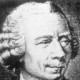## The number 2009

by

Inspired in part by the legendary Hardy-Ramanujan anecdote as well as the post “What is interesting about the number 2009?” at  Walking Randomly, I offer a few arithmetic and demographic curiosities.

2009 is odd, which in particular guarantees it can be written as a difference of squares, most obviously as$1005^2 - 1004^2$, but perhaps more interestingly as$45^2 - 4^2$.

Being a difference of squares is equivalent to being a sum of consecutive odd numbers (in the first instance “2009”, in the second instance “9+11+13+…+89”);  some other curious sums that lead to our new friend 2009 include$\frac{1}{15} + \frac{2}{15} + \frac{3}{15} + \cdots + \frac{245}{15}$$\frac{1}{305} + \frac{4}{305} + \frac{9}{305} + \cdots + \frac{ 122^2}{305}$$\frac{1}{1004} + \frac{2}{1004} + \frac{3}{1004}+\cdots +\frac{2008}{1004}$$\frac{1}{1005}+\frac{2}{1005}+\frac{3}{1005}+\cdots+\frac{2009}{1005}$

Hmmm.  There are our friends 1004 and 1005 again!  Interesting….  [See below for conceptual continuity]

2009 can be written as$7^2 \cdot 41$, which among other things guarantees that all groups of order 2009 are solvable.

Based on data published by Statistics Finland, the 2009th largest city in the world has a population of 207,000 people [a three-way tie between São Leopoldo Brazil,  Ashdod Israel, and Lutsk Ukraine].

Forbes famously publishes lists of the top n individuals, companies, etc… in terms of wealth, corporate size, market share, etc…  Forbes’ list of the world’s billionaires has 1125 entries, so we can’t identify the 2009th wealthiest individual.  However, we can use their data to extrapolate and estimate the net worth of the 2009th richest person.The Forbes data has a very good fit to a power law distribution (in the graph, the blue rhombi squares represent individual data points, the black line the power law of best fit).  This model predicts that the 2009th wealthiest individual will have a net worth of$279.27 (2009)^{-0.775} \approx 0.770$ billion dollars, or \$770 million.

Their list of the world’s largest companies, alas, is the “Forbes Global 2000”, and as one might guess, has only 2000 corporations listed.  Unfortunately, Forbes publishes the top 2000 companies as measured by their own internal (unpublished) index, a weighted average of some sort based on sales, profit, assets, market value, etc….  Without knowing how their index is calculated, one can’t easily extrapolate to determine the size of a hypothetical 2009th largest company in the world.  Presumably it is similar to Mitsubishi Gas Chemical, the company ranked 2000th by Forbes in their list.

If these notes inspire you to find more instances or properties of 2009, add them to the comments at “What is interesting about the number 2009?” at  Walking Randomly.

May 2009 bring all of our readers a wonderful new year!

Added 1/1/09: I knew there had to be a rhyme and reason to the recurrence of 1004 and 1005 in both the difference of squares and the sum of fractions deal.  Here’s the scoop:

If x is odd, then$x = \left( \frac{x+1}{2} \right)^2 - \left( \frac{x-1}{2} \right)^2$.

Furthermore,$1 + 2 + \cdots + (x-1) = \frac{ (x-1)x}{2}$, so in fact$\frac{1}{(x-1)/2} + \frac{2}{(x-1)/2} + \cdots + \frac{x-1}{(x-1)/2} = x$,

and similarly since$1+2+\cdots +x = \frac{x(x+1)}{2}$, we also have$\frac{1}{(x+1)/2} + \frac{2}{(x+1)/2} + \cdots + \frac{x}{(x+1)/2} = x$.

### 4 Responses to “The number 2009”

1.loto no.? Says:

do you have loto no based on 2009 stat?

2.TwoPi Says:

I’m not a lotto-oriented person. Most lotteries pay out roughly 50% of the money wagered, which is by far the largest house cut of any form of gambling. So playing the lotto doesn’t make financial sense.

However, if you get enough enjoyment out of playing lotto, you might find the expense justifiable when compared to the costs of other forms of entertainment (books, movies, travel, opera, etc…).

From the entertainment point of view, you should pick your own favorite numbers, or perhaps contrive some personal algorithm that will give you joy as you use it to select your numbers for the week. (E.g., pick your six favorite companies, and use their stock’s trading price to choose numbers….)

A strategy to maximize your payout in the off chance that you win the lotto: be sure to pick at least one number larger than 31. Since a considerable number of lotto players chose their numbers based on significant dates in their lives, many lotto players will have all their numbers range from 1 to 31. By having at least one number bigger than 31, you increase the likelihood that if you win, you won’t have to share the payout with anyone else.

For me, though, the bottom line is that the only way to reliably win at the lottery, is to run the lottery. (Or to be a taxpayer in a state with a lottery, and never play it.)

3. The 2009 Mathematics Game « Let’s Play Math! Says:

[…] Check out Happy 41*7^2, What is interesting about the number 2009?, and The number 2009. […]

4. Carnival of Mathematics 47, where no, well… « JD2718 Says:

[…] Denise at Let’s Play Math celebrates the new year with the 2009 game. Mike at Walking Randomly explores “What is interesting (mathematically) about 2009” And twopi at 360 (come one, you have to smile for that, right?) has both interesting and trivial exploration of the number 2009. […]